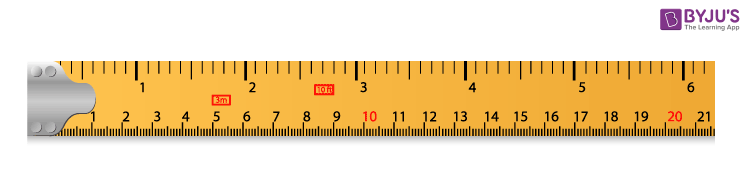# Measurement

Measurement is the basic concept in the study of Mathematics and Science. Measurement quantifies the characteristics of an object or event, which we can compare with other things or events. Measurement is the most commonly used word, whenever we deal with the division of a quantity. Also, in taking a certain amount of things to accomplish a particular task. In our daily existence, we often come across different measurement types for length, weight, times, etc. In this article, you will learn the meaning and definitions of measurement, along with types of measurement and examples.

## Measurement Definition

Measurement is a technique in which the properties of an object are determined by comparing them to a standard quantity. Also, measurement is the essential metric to express any quantity of objects, things and events.

## Types of Measurement

There are some basic types of measurement variables that we deal with in several areas of mathematics. They are:

• Time
• Length
• Weight
• Volume
• Temperature

### Measurement Units

We have units of measurement for different types of measurement as mentioned above. Let’s have a look at the below units of measurement.

Time: Units for expressing time include seconds, minutes, hours, days, weeks, months, years, etc.

Length: Units for measuring length include millimetres, centimetres, meters, kilometres, etc.

Weight: Units for expressing the weight of certain objects include grams, kilograms, tons, etc.

Volume: Units for expressing volume include cm3, m3, litres, etc.

Temperature: The major units of temperature include centigrade and fahrenheit.

It is also possible to perform the conversion of units from one unit to another.

### Measurement Instruments

Instruments are required to measure any type of quantity. For example, to measure weights we require a weighing machine, to measure length we require a scale or tape. Some measurements are given below:

Measurement Tape

The below figure shows the measurement tape that can be used to measure the length of objects or distances.An instrument for measuring time

The pendulum clock is one of the instruments that can be used to measure time.Instruments that measure weightAlso, try: Measurement Calculator

### Measurement and Scaling

We have different scales of measurement such as nominal, ordinal, interval and ratio scale. Let’s understand these measurement scales in brief.

Nominal measurement: The nominal measurement scale is the first level of measurement scale in which the numbers serve as titles or labels to categorise or recognise the objects. This scaling usually deals with non-numeric variables or objects with no value.

Ordinal measurement: The ordinal measurement scale is the second level of measurement that declares the ordering and ranking of data without designating the degree of variation among them. Ordinal symbolises the “order.” Ordinal data is referred to as qualitative or categorical data, and this data can be grouped and named also can be ranked.

Interval measurement: The interval measurement scale is the third level of measurement scale. This scaling is a quantitative measurement scale in which we can express the difference between any two variables.

Ratio measurement: The ratio measurement scale is the fourth level of measurement scale, which is quantitative. The ratio scaling has a unique feature: it possesses the origin or zero points character.

### Applications of Measurement

Measurement is an important concept for the following situations.

• To construct buildings, we need to consider proper dimensions.